xTime-of-flight mass spectrometryEncyclopedia
Time-of-flight mass spectrometry (TOFMS) is a method of mass spectrometry
Mass spectrometry
Mass spectrometry is an analytical technique that measures the mass-to-charge ratio of charged particles.It is used for determining masses of particles, for determining the elemental composition of a sample or molecule, and for elucidating the chemical structures of molecules, such as peptides and...

in which an ion
Ion
An ion is an atom or molecule in which the total number of electrons is not equal to the total number of protons, giving it a net positive or negative electrical charge. The name was given by physicist Michael Faraday for the substances that allow a current to pass between electrodes in a...

's mass-to-charge ratio
Mass-to-charge ratio
The mass-to-charge ratio ratio is a physical quantity that is widely used in the electrodynamics of charged particles, e.g. in electron optics and ion optics. It appears in the scientific fields of lithography, electron microscopy, cathode ray tubes, accelerator physics, nuclear physics, Auger...

is determined via a time measurement. Ions are accelerated by an electric field
Electric field
In physics, an electric field surrounds electrically charged particles and time-varying magnetic fields. The electric field depicts the force exerted on other electrically charged objects by the electrically charged particle the field is surrounding...

of known strength. This acceleration results in an ion having the same kinetic energy
Kinetic energy
The kinetic energy of an object is the energy which it possesses due to its motion.It is defined as the work needed to accelerate a body of a given mass from rest to its stated velocity. Having gained this energy during its acceleration, the body maintains this kinetic energy unless its speed changes...

as any other ion that has the same charge. The velocity of the ion depends on the mass-to-charge ratio
Mass-to-charge ratio
The mass-to-charge ratio ratio is a physical quantity that is widely used in the electrodynamics of charged particles, e.g. in electron optics and ion optics. It appears in the scientific fields of lithography, electron microscopy, cathode ray tubes, accelerator physics, nuclear physics, Auger...

. The time that it subsequently takes for the particle to reach a detector at a known distance is measured. This time will depend on the mass-to-charge ratio of the particle (heavier particles reach lower speeds). From this time and the known experimental parameters one can find the mass-to-charge ratio of the ion.

## Theory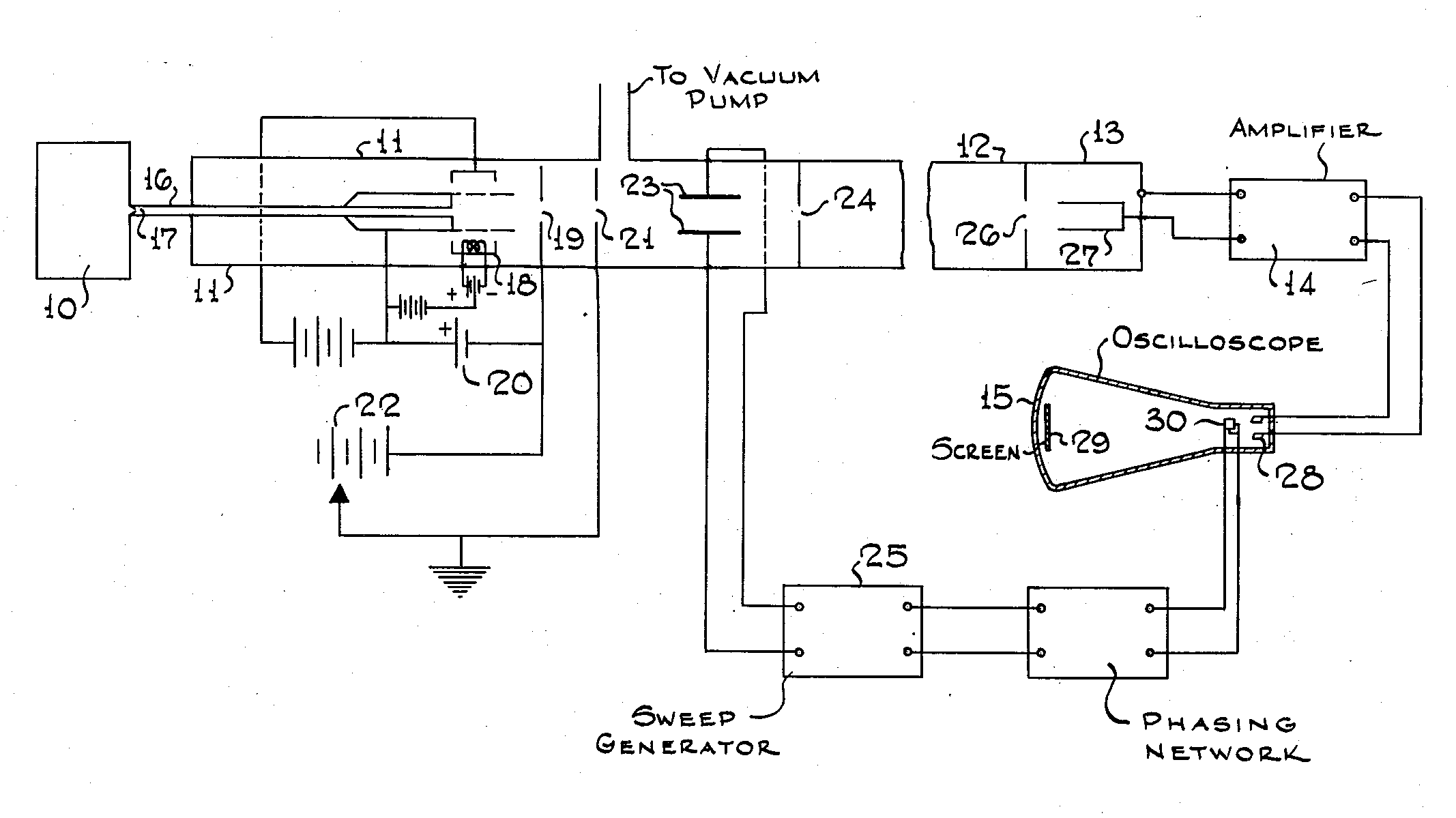The potential energy
Potential energy
In physics, potential energy is the energy stored in a body or in a system due to its position in a force field or due to its configuration. The SI unit of measure for energy and work is the Joule...

of a charged particle in an electric field is related to the charge of the particle and to the strength of the electric field: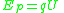where Ep is potential energy, q is the charge of the particle, and U is the electric potential difference (also known as voltage).

When the charged particle is accelerated into time-of-flight tube by the voltage U, its potential energy is converted to kinetic energy
Kinetic energy
The kinetic energy of an object is the energy which it possesses due to its motion.It is defined as the work needed to accelerate a body of a given mass from rest to its stated velocity. Having gained this energy during its acceleration, the body maintains this kinetic energy unless its speed changes...

. The kinetic energy of any mass
Mass
Mass can be defined as a quantitive measure of the resistance an object has to change in its velocity.In physics, mass commonly refers to any of the following three properties of matter, which have been shown experimentally to be equivalent:...

is: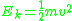In effect, the potential energy is converted to kinetic energy, meaning that equations  and  are equal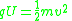The velocity
Velocity
In physics, velocity is speed in a given direction. Speed describes only how fast an object is moving, whereas velocity gives both the speed and direction of the object's motion. To have a constant velocity, an object must have a constant speed and motion in a constant direction. Constant ...

of the charged particle after acceleration will not change since it moves in a field-free time-of-flight tube. The velocity of the particle can be determined in a time-of-flight tube since the length of the path (d) of the flight of the ion is known and the time of the flight of the ion (t) can be measured using a transient digitizer or time to digital converter
Time to digital converter
In electronic instrumentation and signal processing, a time to digital converter is a device for converting a signal of sporadic pulses into a digital representation of their time indices. In other words, a TDC outputs the time of arrival for each incoming pulse...

.

Thus,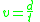and we substitute
Elementary algebra
Elementary algebra is a fundamental and relatively basic form of algebra taught to students who are presumed to have little or no formal knowledge of mathematics beyond arithmetic. It is typically taught in secondary school under the term algebra. The major difference between algebra and...

the value of v in Eqn  into Eqn .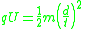Rearranging Eqn  so that the flight time is expressed by everything else: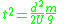Taking the square root
Square root
In mathematics, a square root of a number x is a number r such that r2 = x, or, in other words, a number r whose square is x...

of the timeThese factors for the time of flight have been grouped purposely.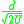contains constant
Constant (mathematics)
In mathematics, a constant is a non-varying value, i.e. completely fixed or fixed in the context of use. The term usually occurs in opposition to variable In mathematics, a constant is a non-varying value, i.e. completely fixed or fixed in the context of use. The term usually occurs in opposition...

s that in principle do not change when a set of ions are analyzed in a single pulse of acceleration
Acceleration
In physics, acceleration is the rate of change of velocity with time. In one dimension, acceleration is the rate at which something speeds up or slows down. However, since velocity is a vector, acceleration describes the rate of change of both the magnitude and the direction of velocity. ...

. Eqn 8 can thus be given as: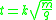where k is a proportionality constant
Proportionality (mathematics)
In mathematics, two variable quantities are proportional if one of them is always the product of the other and a constant quantity, called the coefficient of proportionality or proportionality constant. In other words, are proportional if the ratio \tfrac yx is constant. We also say that one...

representing factors related to the instrument settings and characteristics.

Eqn  reveals more clearly that the time of flight of the ion varies with the square root
Square root
In mathematics, a square root of a number x is a number r such that r2 = x, or, in other words, a number r whose square is x...

of its mass-to-charge ratio
Mass-to-charge ratio
The mass-to-charge ratio ratio is a physical quantity that is widely used in the electrodynamics of charged particles, e.g. in electron optics and ion optics. It appears in the scientific fields of lithography, electron microscopy, cathode ray tubes, accelerator physics, nuclear physics, Auger...

(m/q).

Consider a real world example of a MALDI time-of-flight mass spectrometer instrument which is used to produce a mass spectrum
Mass spectrum
A mass spectrum is an intensity vs. m/z plot representing a chemical analysis. Hence, the mass spectrum of a sample is a pattern representing the distribution of ions by mass in a sample. It is a histogram usually acquired using an instrument called a mass spectrometer...

of the tryptic peptides of a protein
Protein
Proteins are biochemical compounds consisting of one or more polypeptides typically folded into a globular or fibrous form, facilitating a biological function. A polypeptide is a single linear polymer chain of amino acids bonded together by peptide bonds between the carboxyl and amino groups of...

. Suppose the mass of one tryptic peptide is 1000 daltons (Da
Atomic mass unit
The unified atomic mass unit or dalton is a unit that is used for indicating mass on an atomic or molecular scale. It is defined as one twelfth of the rest mass of an unbound neutral atom of carbon-12 in its nuclear and electronic ground state, and has a value of...

). The kind of ionization
Ionization
Ionization is the process of converting an atom or molecule into an ion by adding or removing charged particles such as electrons or other ions. This is often confused with dissociation. A substance may dissociate without necessarily producing ions. As an example, the molecules of table sugar...

of peptides produced by MALDI is typically +1 ions, so q = e
Elementary charge
The elementary charge, usually denoted as e, is the electric charge carried by a single proton, or equivalently, the absolute value of the electric charge carried by a single electron. This elementary charge is a fundamental physical constant. To avoid confusion over its sign, e is sometimes called...

in both cases. Suppose the instrument is set to accelerate the ions in a U = 15,000 volt
Volt
The volt is the SI derived unit for electric potential, electric potential difference, and electromotive force. The volt is named in honor of the Italian physicist Alessandro Volta , who invented the voltaic pile, possibly the first chemical battery.- Definition :A single volt is defined as the...

s (15 kilovolt, or 15 kV) potential. And suppose the length of the flight tube is 1.5 meters (typical). All the factors necessary to calculate the time of flight of the ions are now known for Eqn , which is evaluated first of the ion of mass 1000 Da:Note that the mass had to be converted from daltons (Da) to kilogram
Kilogram
The kilogram or kilogramme , also known as the kilo, is the base unit of mass in the International System of Units and is defined as being equal to the mass of the International Prototype Kilogram , which is almost exactly equal to the mass of one liter of water...

s (kg) to make it possible to evaluate the equation in the proper units. The final value should be in seconds: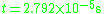Microsecond
A microsecond is an SI unit of time equal to one millionth of a second. Its symbol is µs.A microsecond is equal to 1000 nanoseconds or 1/1000 millisecond...

s. If there were a singly charged tryptic peptide
Peptide
Peptides are short polymers of amino acid monomers linked by peptide bonds. They are distinguished from proteins on the basis of size, typically containing less than 50 monomer units. The shortest peptides are dipeptides, consisting of two amino acids joined by a single peptide bond...

ion with 4000 Da mass, and it is four times larger than the 1000 Da mass, it would take twice the time, or about 56 microseconds to traverse the flight tube, since time is proportional
Proportionality (mathematics)
In mathematics, two variable quantities are proportional if one of them is always the product of the other and a constant quantity, called the coefficient of proportionality or proportionality constant. In other words, are proportional if the ratio \tfrac yx is constant. We also say that one...

to the square root
Square root
In mathematics, a square root of a number x is a number r such that r2 = x, or, in other words, a number r whose square is x...

of the mass-to-charge ratio.

## Delayed extraction

Mass resolution can be improved in axial MALDI-TOF mass spectrometer where ion production takes place in vacuum by allowing the initial burst of ions and neutrals produced by the laser pulse to equilibrate and to let the ions travel some distance perpendicularly to the sample plate before the ions can be accelerated into the flight tube. The ion equilibration in plasma plume produced during the desorption/ionization takes place approximately 100 ns or less, after that most of ions irrespectively of their mass start moving from the surface with some average velocity. To compensate for the spread of this average velocity and to improve mass resolution, it was proposed to delay the extraction of ions from the ion source toward the flight tube by a few hundred nanoseconds to a few microseconds with respect to the start of short (typically, a few nanosecond) laser pulse. This technique is referred to as "time-lag focusing" for ionization of atoms or molecules by Resonance enhanced multiphoton ionization or by electron impact ionization in a rarefied gas and "delayed extraction" for ions produced generally by laser desorption/ionization of molecules adsorbed on flat surfaces or microcrystals placed on conductive flat surface.

Delayed extraction generally refers to the operation mode of vacuum ion sources when the onset of the electric field responsible for acceleration (extraction) of the ions into the flight tube is delayed by some short time (200-500 ns)with respect to the ionization (or desorption/ionization) event. This differs from a case of constant extraction field where the ions are accelerated instantaneously upon being formed. Delayed extraction is used with MALDI or laser desorption/ionization (LDI) ion sources where the ions to be analyzed are produced in an expanding plume moving from the sample plate with a high speed (400–1000 m/s). Since the thickness of the ion packets arriving at the detector is important to mass resolution, on first inspection it can appear counter-intuitive to allow the ion plume to further expand before extraction. Delayed extraction is more of a compensation for the initial momentum of the ions: it provides the same arrival times at the detector for ions with the same mass-to-charge ratios but with different initial velocities.

In delayed extraction of ions produced in vacuum, the ions that have lower momentum in the direction of extraction start to be accelerated at higher potential due to being further from the extraction plate when the extraction field is turned on. Conversely, those ions with greater forward momentum start to be accelerated at lower potential since they are closer to the extraction plate. At the exit from the acceleration region, the slower ions at the back of the plume will be accelerated to greater velocity than the initially faster ions at the front of the plume. So after delayed extraction, a group of ions that leaves the ion source earlier has lower velocity in the direction of the acceleration compared to some other group of ions that leaves the ion source later but with greater velocity. When ion source parameters are properly adjusted, the faster group of ions catches up the slower one at some distance from the ion source, so the detector plate placed at this distance detects simultaneous arrival of these groups of ions. In its way, the delayed application of the acceleration field acts as a one-dimensional time-of-flight focusing element.

## Reflectron TOF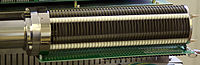The kinetic energy distribution in the direction of ion flight can be corrected by using a reflectron. The reflectron
Reflectron
A reflectron is a type of time-of-flight mass spectrometer that comprises a pulsed ion source, field-free region, ion mirror, and ion detector and uses a static or time dependent electric field in the ion mirror to reverse the direction of travel of the ions entering it...

uses a constant electrostatic field to reflect the ion beam toward the detector. The more energetic ions penetrate deeper into the reflectron, and take a slightly longer path to the detector. Less energetic ions of the same mass-to-charge ratio penetrate a shorter distance into the reflectron and, correspondingly, take a shorter path to the detector. The flat surface of the ion detector (typically a microchannel plate, MCP) is placed at the point where ions with different energies reflected by the reflectron hit a surface of the detector at the same time counted with respect to the onset of the extraction pulse in the ion source. A point of simultaneous arrival of ions of the same mass and charge but with different energies is often referred as time-of-flight focus.
An additional advantage to the re-TOF arrangement is that twice the flight path is achieved in a given length of instrument.

## Ion gating

A Bradbury–Nielsen shutter is a type of ion gate used in TOF mass spectrometers and in ion mobility spectrometer
Ion mobility spectrometer
Ion-mobility spectrometry is an analytical technique used to separate and identify ionized molecules in the gas phase based on their mobility in a carrier buffer gas...

s, as well as Hadamard transform
The Hadamard transform is an example of a generalized class of Fourier transforms...

TOF mass spectrometers. The Bradbury–Nielsen shutter is ideal for fast timed ion selector (TIS)—a device used for isolating ions over narrow mass range in tandem (TOF/TOF) MALDI mass spectrometers.

## Orthogonal acceleration time-of-flight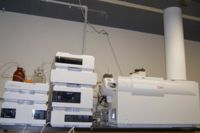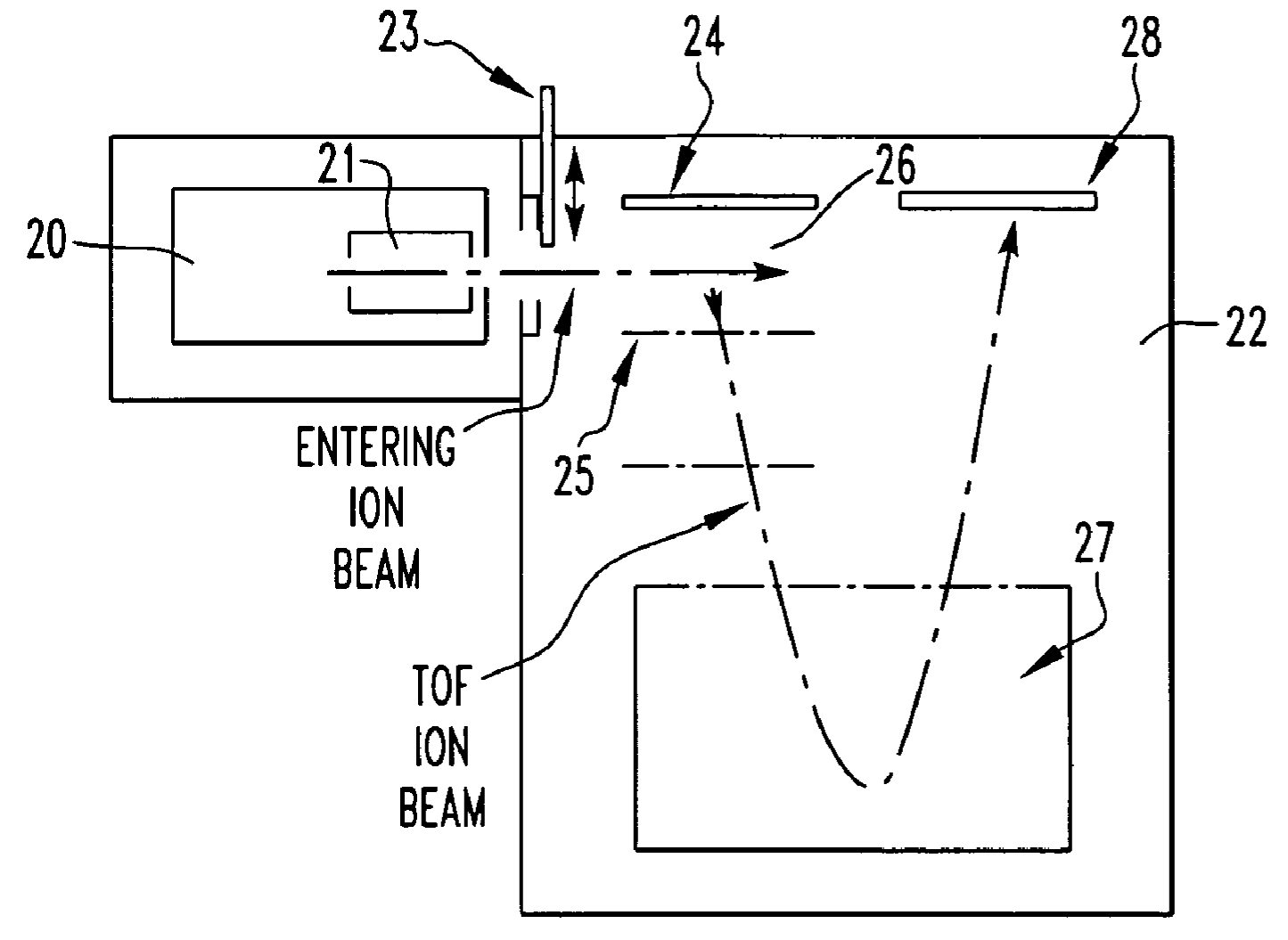Continuous ion sources (most commonly electrospray ionization, ESI) are generally interfaced to the TOF mass analyzer by "orthogonal extraction" in which ions introduced into the TOF mass analyzer are accelerated along the axis perpendicular to their initial direction of motion. Orthogonal acceleration combined with collisional ion cooling allows separating the ion production in the ion source and mass analysis. In this technique, very high resolution can be achieved for ions produced in MALDI or ESI sources.
Before entering the orthogonal acceleration region or the pulser, the ions produced in continuous (ESI) or pulsed (MALDI) sources are focused (cooled) into a beam of 1–2 mm diameter by collisions with a residual gas in RF multipole guides. A system of electrostatic lenses mounted in high-vacuum region before the pulser makes the beam parallel to minimize its divergence in the direction of acceleration. The combination of ion collisional cooling and orthogonal acceleration TOF has provided significant increase in resolution of modern TOF MS from few hundred to several tens of thousand without compromising the sensitivity.

## Hadamard transform time-of-flight mass spectrometry

The Hadamard transform is an example of a generalized class of Fourier transforms...

TOF mass spectrometer is an instrument that can be used with a continuous ion source. Whereas traditional TOF MS analyzes one packet of ions at a time, waiting for the ions to reach the detector before introducing another ion packet, HT-TOFMS can analyze several ion packets simultaneously traveling in the flight tube. The ions packets are encoded by fast modulating the transmission of the ion beam in a manner based on a Hadamard matrix
In mathematics, an Hadamard matrix, named after the French mathematician Jacques Hadamard, is a square matrix whose entries are either +1 or −1 and whose rows are mutually orthogonal...

. The resulting spectra,however, can be deconvoluted based on knowledge of the encoding scheme.

## Tandem TOF/TOF

TOF/TOF is a tandem mass spectrometry
Tandem mass spectrometry
Tandem mass spectrometry, also known as MS/MS or MS2, involves multiple steps of mass spectrometry selection, with some form of fragmentation occurring in between the stages.-Tandem MS instruments:...

method where two time-of-flight mass spectrometers are used consecutively. To record full spectrum of precursor (parent) ions TOF/TOF operates in MS mode. In this mode, the energy of the pulse laser is chosen slightly above the onset of MALDI for specific matrix in use to ensure the compromise between an ion yield for all the parent ions and reduced fragmentation of the same ions. When operating in a tandem (MS/MS) mode, the laser energy is increased considerably above MALDI threshold. The first TOF mass spectrometer (basically, a flight tube which ends up with the timed ion selector) isolates precursor ions of choice using a velocity filter, typically, of a Bradbury-Nielsen type, and the second TOF-MS (that includes the post accelerator, flight tube, ion mirror, and the ion detector) analyzes the fragment ions. Fragment ions in MALDI TOF/TOF result from decay of precursor ions vibrationally excited above their dissociation level in MALDI source (post source decay ). Additional ion fragmentation implemented in a high-energy collision cell may be added to the system to increase dissociation rate of vibrationally excited precursor ions. Some designs include precursor signal quenchers as a part of second TOF-MS to reduce the instant current load on the ion detector.

## Detectors

A time-of-flight mass spectrometer (TOFMS) consists of a mass analyzer and a detector. An ion source (either pulsed or continuous) is used for lab-related TOF experiments, but not needed for TOF analyzers used in space, where the sun or planetary ionospheres provide the ions. The TOF mass analyzer can be a linear flight tube or a reflectron. The ion detector typically consists of microchannel plate detector
Microchannel plate detector
A micro-channel plate is a planar component used for detection of particles and impinging radiation . It is closely related to an electron multiplier, as both intensify single particles or photons by the multiplication of electrons via secondary emission...

or a fast secondary emission
Secondary emission
Secondary emission in physics is a phenomenon where primary incident particles of sufficient energy, when hitting a surface or passing through some material, induce the emission of secondary particles. The primary particles are often charged particles like electrons or ions. If the secondary...

multiplier (SEM) where first converter plate (dynode
Dynode
A dynode is one of a series of electrodes within a photomultiplier tube. Each dynode is at a more positive electrical potential than its predecessor. Secondary emission occurs at the surface of each dynode...

) is flat. The electrical signal from the detector is recorded by means of a time to digital converter
Time to digital converter
In electronic instrumentation and signal processing, a time to digital converter is a device for converting a signal of sporadic pulses into a digital representation of their time indices. In other words, a TDC outputs the time of arrival for each incoming pulse...

(TDC) or a fast analog-to-digital converter
Analog-to-digital converter
An analog-to-digital converter is a device that converts a continuous quantity to a discrete time digital representation. An ADC may also provide an isolated measurement...

(ADC). TDC is mostly used in combination with orthogonal-acceleration (oa)TOF instruments.

Time-to-digital converters register the arrival of a single ion at discrete time "bins"; a combination of threshold triggering and constant fraction discriminator
Constant fraction discriminator
A constant fraction discriminator is an electronic signal processing device, designed to mimic the mathematical operation of finding a maximum of a pulse by finding the zero of its slope....

(CFD) discriminates between noise and ion arrival events and converts 1-2 nanosecond long Gaussian-shaped electrical pulses of different amplitudes from MCP into common-shape pulses (e.g., pulses compatible with TTL logic circuitry) sent to TDC. Using CFD provides a time point correspondent to a position of peak maximum independent of gain peal amplitude caused by variation of the MCP or SEM. Fast CFDs typically have dead time of 1–2 seconds thus preventing repetitive triggering from the same pulse.

The TDC is an ion counting detector – it can be extremely fast (down to a few picosecond resolution), but its dynamic range
Dynamic range
Dynamic range, abbreviated DR or DNR, is the ratio between the largest and smallest possible values of a changeable quantity, such as in sound and light. It is measured as a ratio, or as a base-10 or base-2 logarithmic value.-Dynamic range and human perception:The human senses of sight and...

is limited due to its inability to properly count the events when more than one ions simultaneously hit the detector. The outcome of limited dynamic range is that the number of ions detected in one spectrum is somewhat small. This problem of limited dynamic range can be alleviated using multichannel detector design: an array of mini-anodes attached to a common MCP stack and multiple TDC, where each TDC records signals from individual mini-anode. To obtain peaks with statistically acceptable intensities, ion counting is accompanied by summing of hundreds of individual mass spectra (so-called hystograming). To reach a very high counting rate (limited only by duration of individual TOF spectrum, ~ 200 microsecond in long or multipath TOF setups), a very high repetition rate of ion extractions to the TOF tube is used. Orthogonal acceleration TOF mass analyzers operate at 20–30 kHz repetition rates. In combined mass spectra obtained by summing a large number of individual ion detection events, each peak is a histogram
Histogram
In statistics, a histogram is a graphical representation showing a visual impression of the distribution of data. It is an estimate of the probability distribution of a continuous variable and was first introduced by Karl Pearson...

obtained by adding up counts in each individual bin. Because the recording of the individual ion arrival with TDC produces only a single time point (e.g., a time "bin" correspondent to the maximum of the electrical pulse produced in a single-ion detection event), the TDC eliminates the fraction of peak width in combined spectra determined by a limited response time of the MCP detector. This propagates into better mass resolution.

Modern ultra-fast 4 GSample/sec analog-to-digital converter digitizes the pulsed ion current from the MCP detector at discrete time intervals (250 picoseconds). Typical 8-bit or 10-bit 4 GHz ADC has much higher dynamic range than the TDC, which allows its usage in MALDI-TOF instruments with its high peak currents. To record fast analog signal from MCP detector one is required to carefully match the impedance of the detector anode with input circuitry of the ADC (preamplifier
Preamplifier
A preamplifier is an electronic amplifier that prepares a small electrical signal for further amplification or processing. A preamplifier is often placed close to the sensor to reduce the effects of noise and interference. It is used to boost the signal strength to drive the cable to the main...

) to minimize "ringing". Mass resolution in mass spectra recorded with ultra-fast ADC can be improved by using MCP detectors with shorter response times.

## Applications

Matrix-assisted laser desorption ionization (MALDI) is a pulsed ionization technique that is readily compatible with TOF MS.

Atom probe
Atom probe
The atom probe is a microscope used in material science that was invented in 1967 by Erwin Wilhelm Müller, J. A. Panitz, and S. Brooks McLane. The atom probe is closely related to the method of Field Ion Microscopy, which is the first microscopic method to achieve atomic resolution, occurring in...

tomography also takes advantage of TOF mass spectrometry.

## History of the field

An early time-of-flight mass spectrometer, named the Velocitron, was reported by A. E. Cameron and D. F. Eggers Jr, working at the Y-12 National Security Complex
Y-12 National Security Complex
The Y-12 National Security Complex is a United States Department of Energy National Nuclear Security Administration facility located in Oak Ridge, Tennessee, near the Oak Ridge National Laboratory...

, in 1948. The idea had been proposed two years earlier, in 1946, by W. E. Stephens of the University of Pennsylvania
University of Pennsylvania
The University of Pennsylvania is a private, Ivy League university located in Philadelphia, Pennsylvania, United States. Penn is the fourth-oldest institution of higher education in the United States,Penn is the fourth-oldest using the founding dates claimed by each institution...

in a Friday afternoon session of a meeting, at the Massachusetts Institute of Technology
Massachusetts Institute of Technology
The Massachusetts Institute of Technology is a private research university located in Cambridge, Massachusetts. MIT has five schools and one college, containing a total of 32 academic departments, with a strong emphasis on scientific and technological education and research.Founded in 1861 in...

, of the American Physical Society
American Physical Society
The American Physical Society is the world's second largest organization of physicists, behind the Deutsche Physikalische Gesellschaft. The Society publishes more than a dozen scientific journals, including the world renowned Physical Review and Physical Review Letters, and organizes more than 20...

.

### Manufacturers

• Agilent
• AB Sciex
• Bruker
Bruker
Bruker is a leading provider of high-performance scientific instruments and solutions for molecular and materials research, as well as for industrial and applied analysis...

• Jeol
JEOL
is a manufacturer of electron microscopes and other scientific instruments. Its headquarters are in Tokyo, Japan, with 25 subsidiaries and two associated companies....

• LECO Corporation
• Perkin-Elmer